Question

Circuits

In this assignment you need to design the control for an automated washing machine in a laundromat.The machine starts when a coin is deposited and an input from the coin sensor received, which will stay on till reset at the end of the cycle. It sequences through the following stages: soak, wash, rinse and spin.There is one timer. You can assume each stage will take the same amount of time. The timer begin sticking as soon as the coin is deposited, generates a signal at the end of the time period, and then resets itself and starts again. If the lid is raised during the spin cycle, the machine stops spinning and goes to the end of the cycle, with the timer reset also. At the end of the cycle the timer and the coin sensor will need to be reset ready for the next user.

a) Identify the system inputs and outputs.

b) Draw a state diagram for the FSM.

c) Determine if the number of states can be reduced and assign them with binary codes.

d) Design and implement the FSM using D, T and JK flip-flops.

e) Verify the JK flip-flop implementation in (d).

f) Write Verilog HDL models for the machine based on the state diagram in (b) and the JK flip-flop sequential circuit that you implement in (d).Verified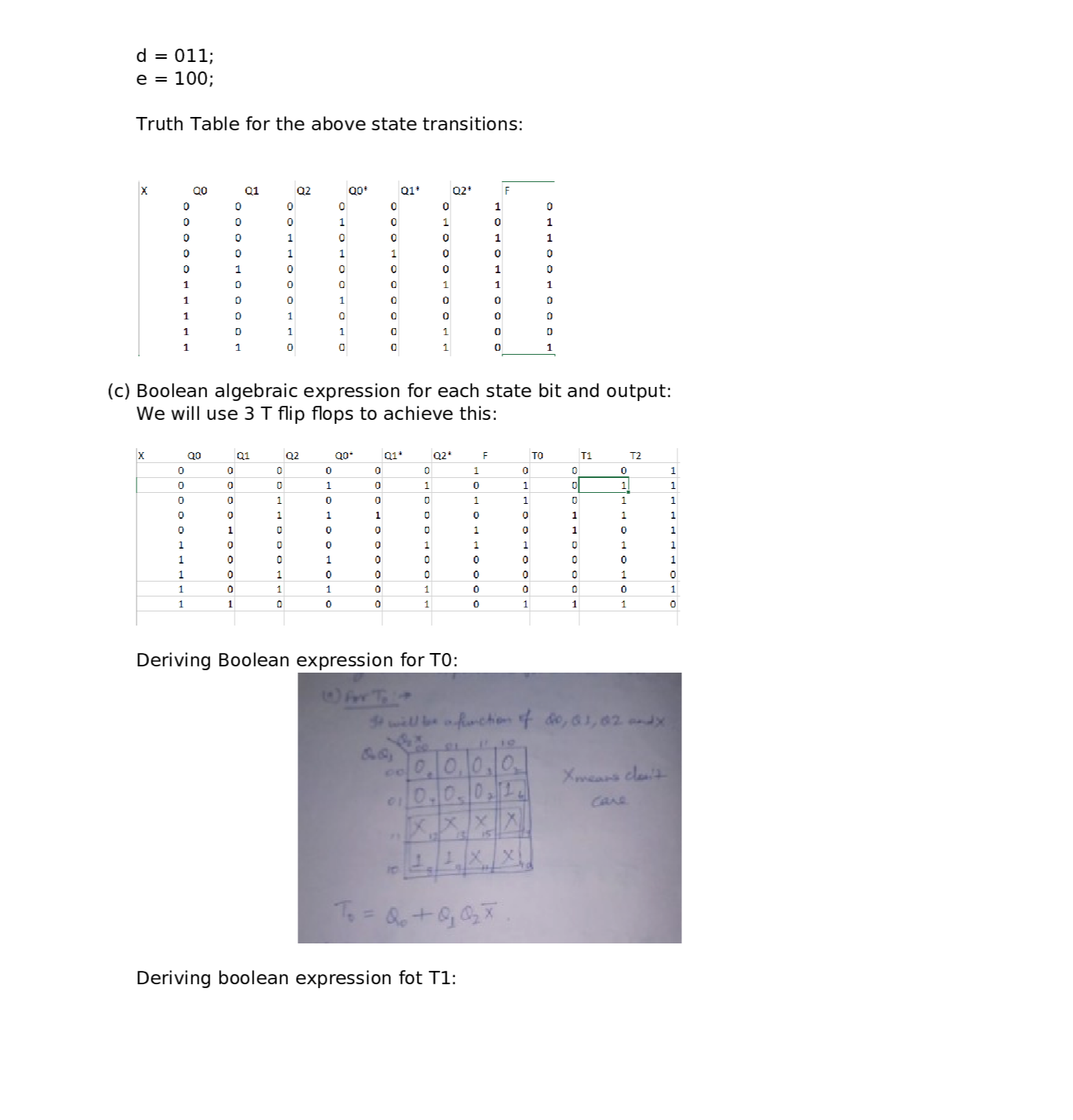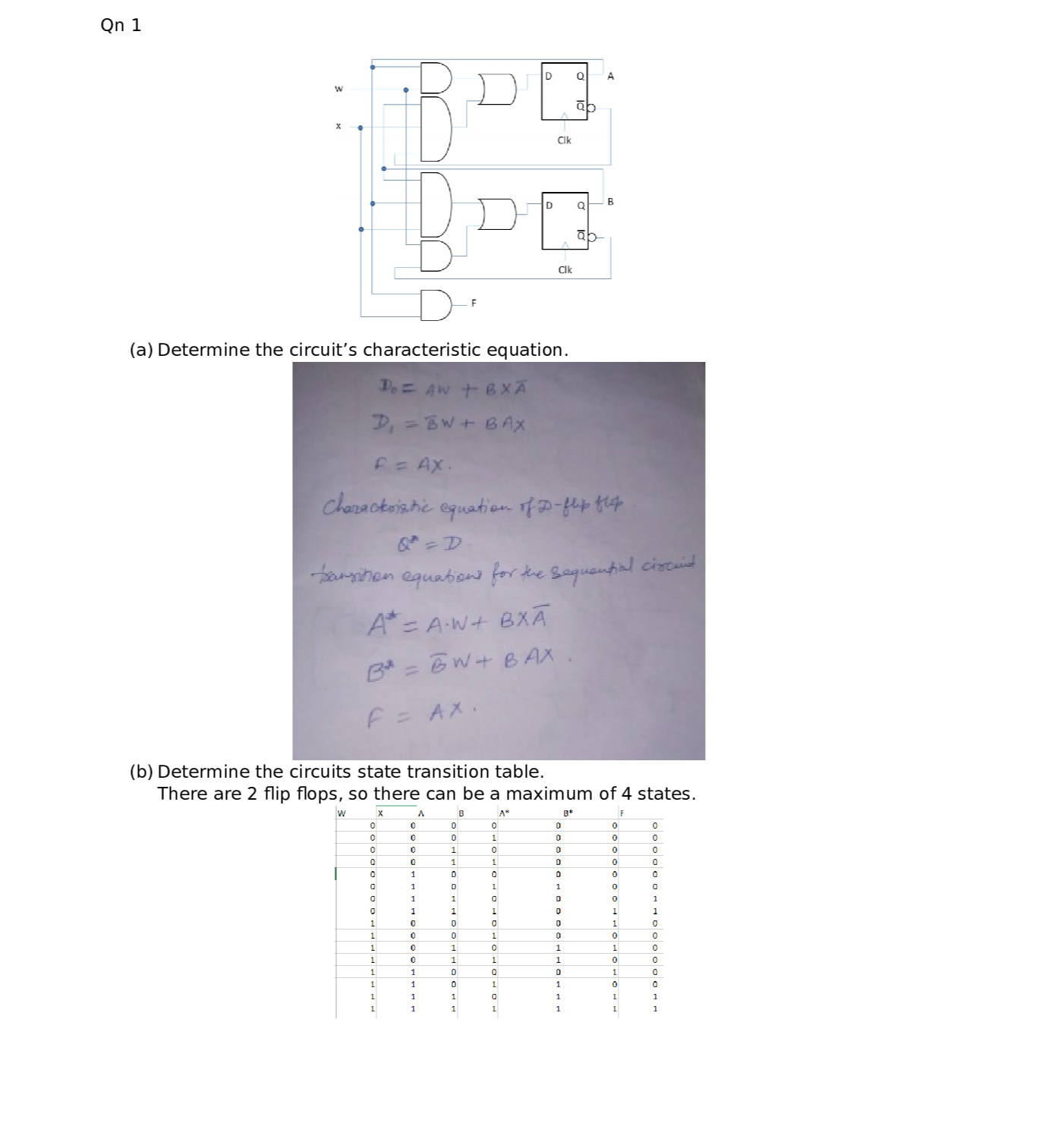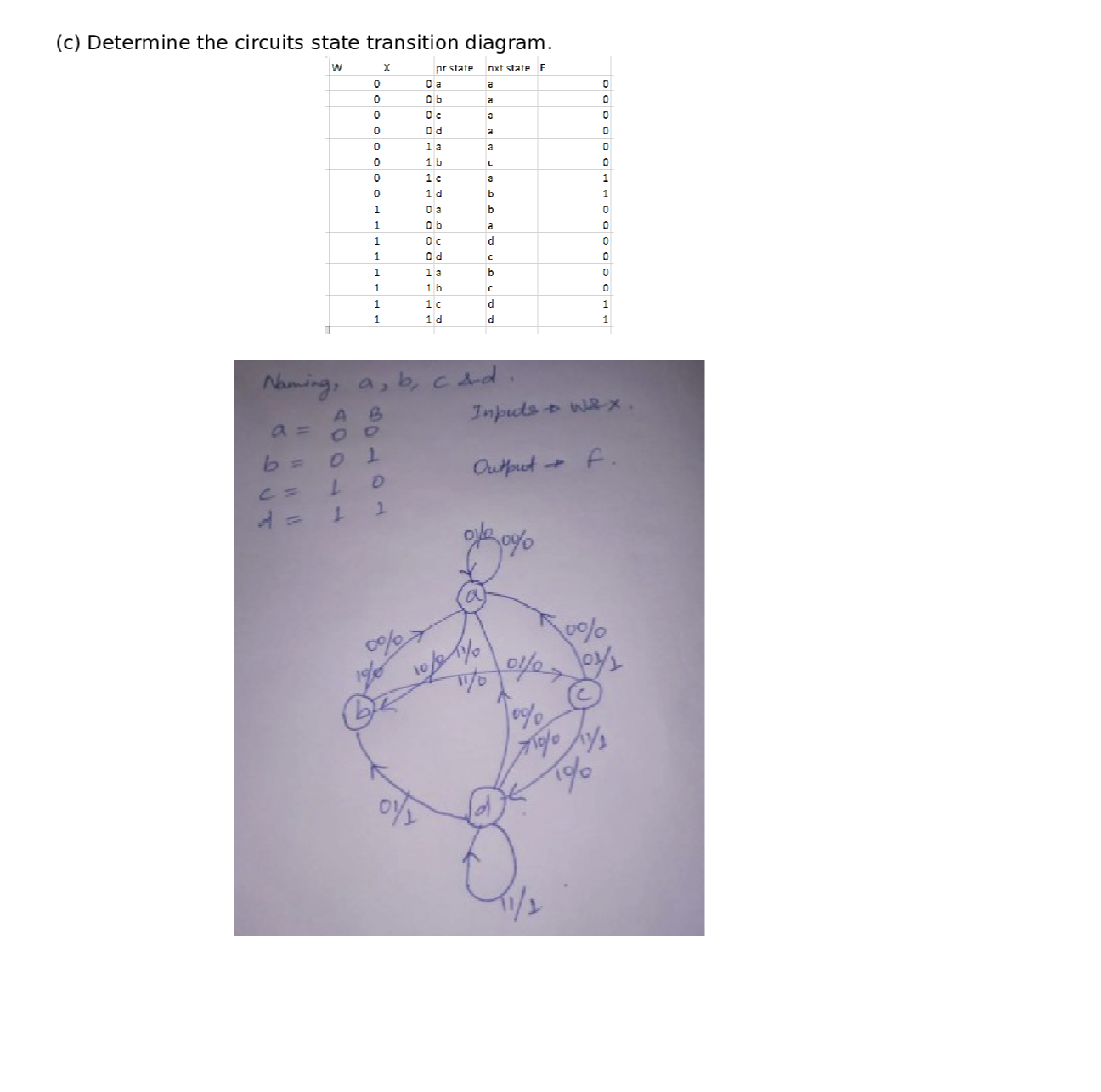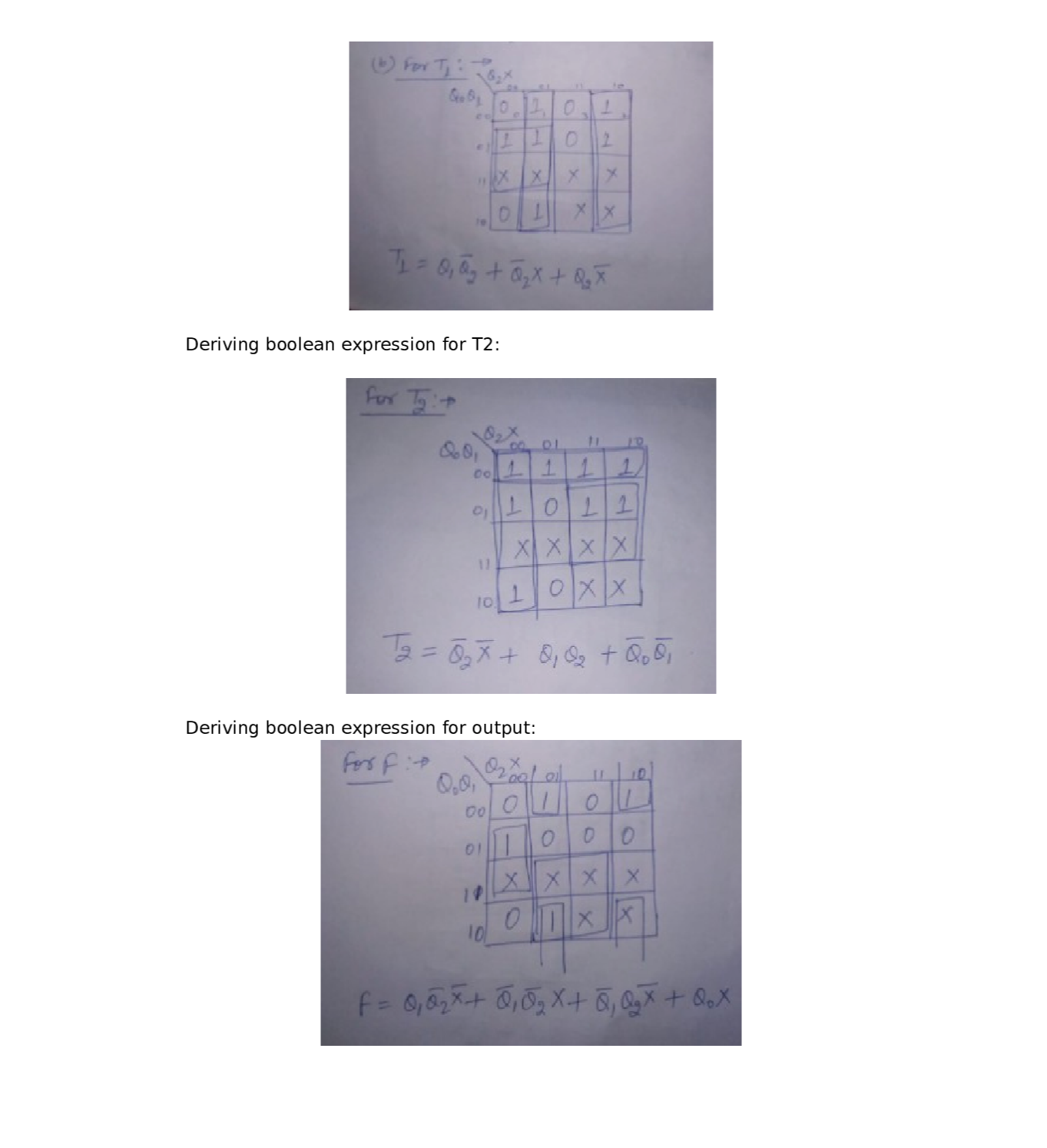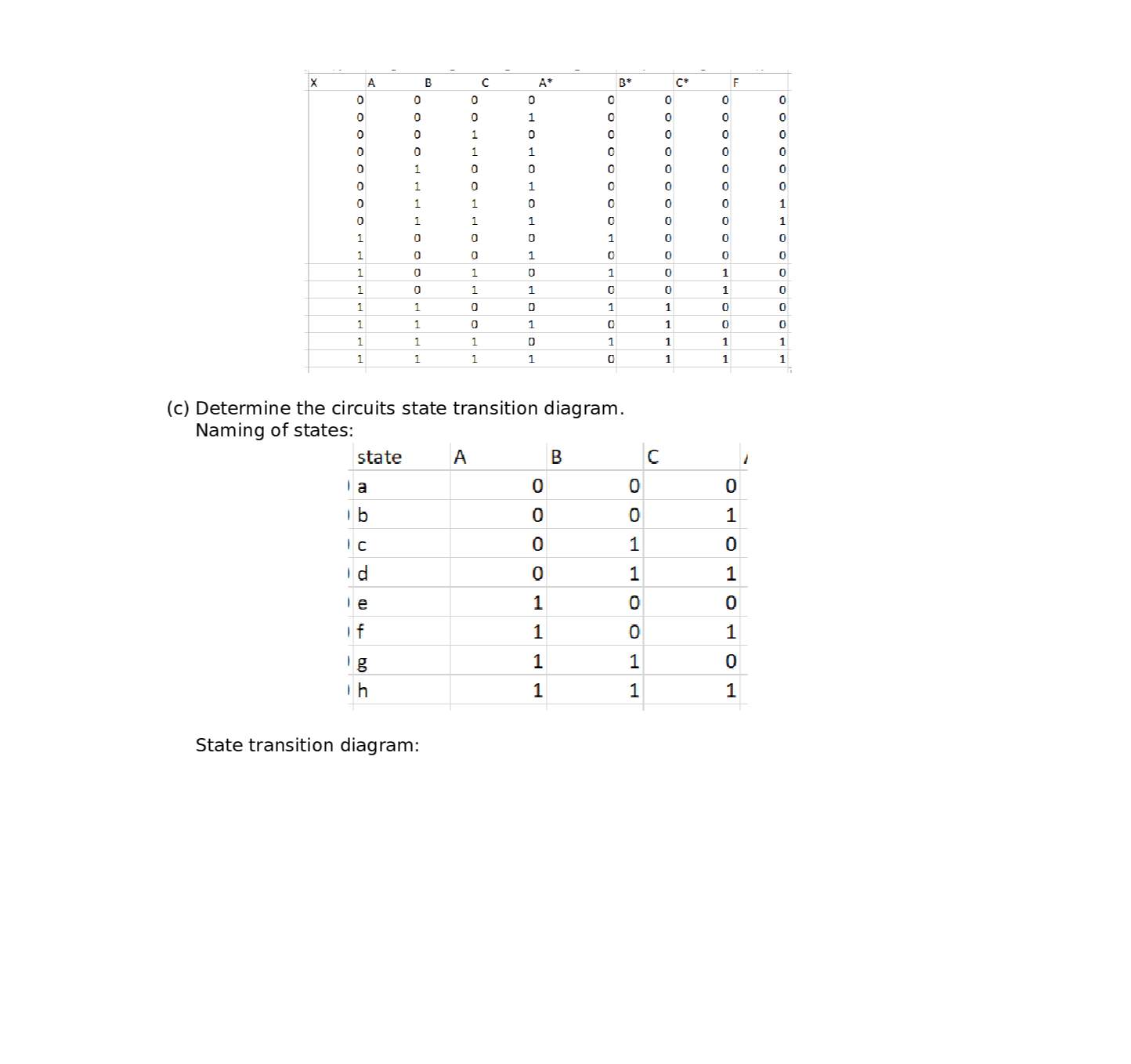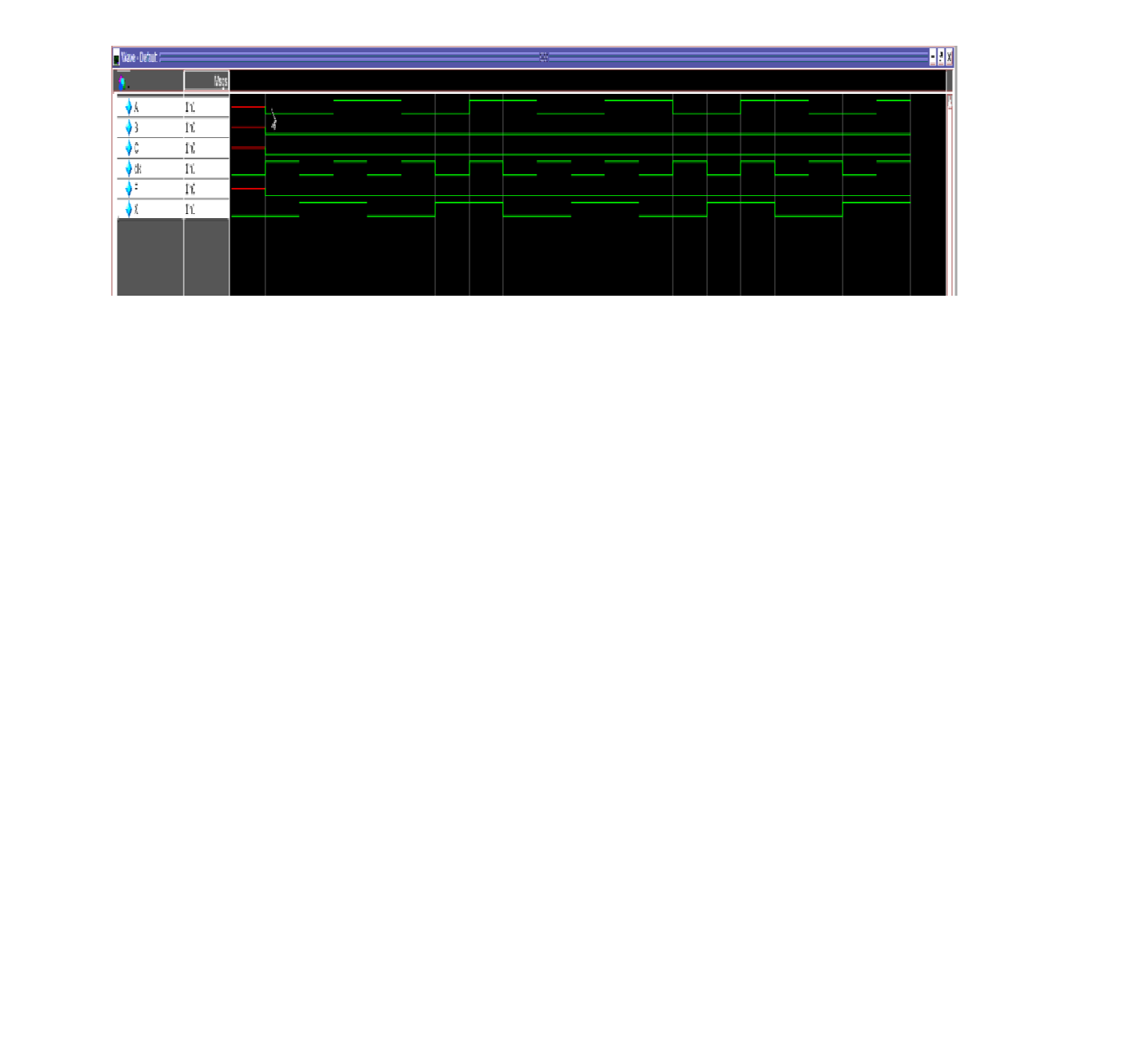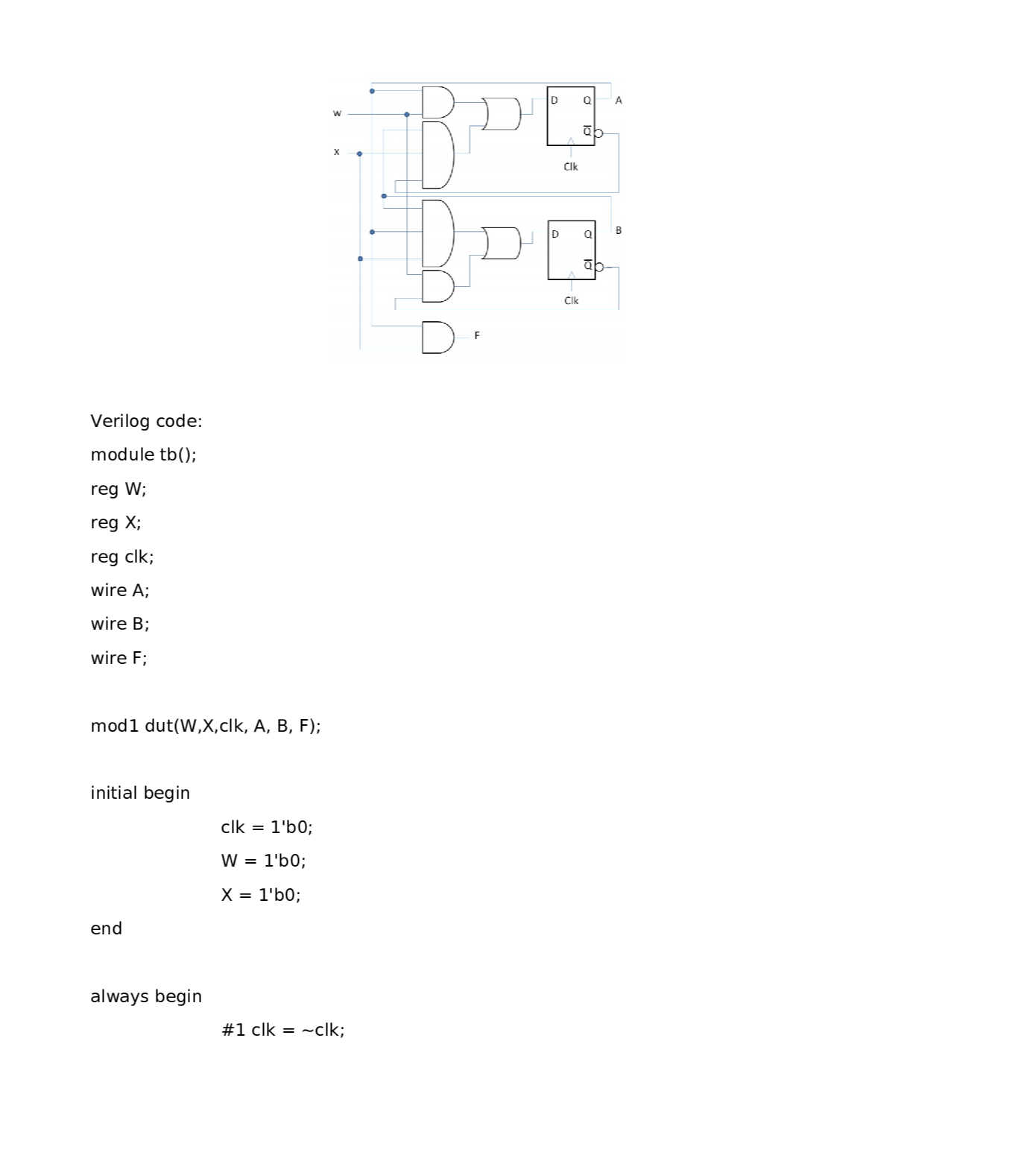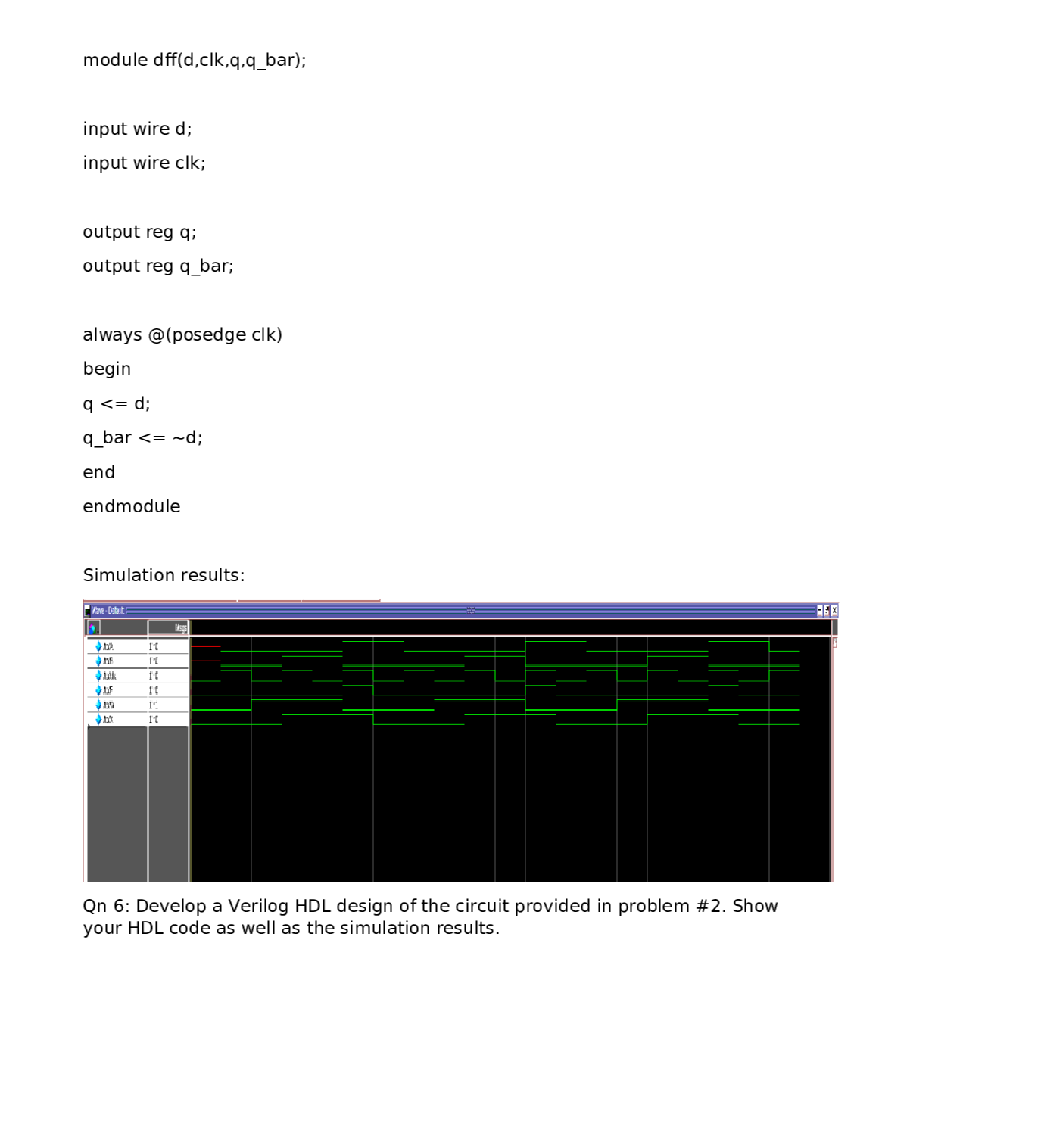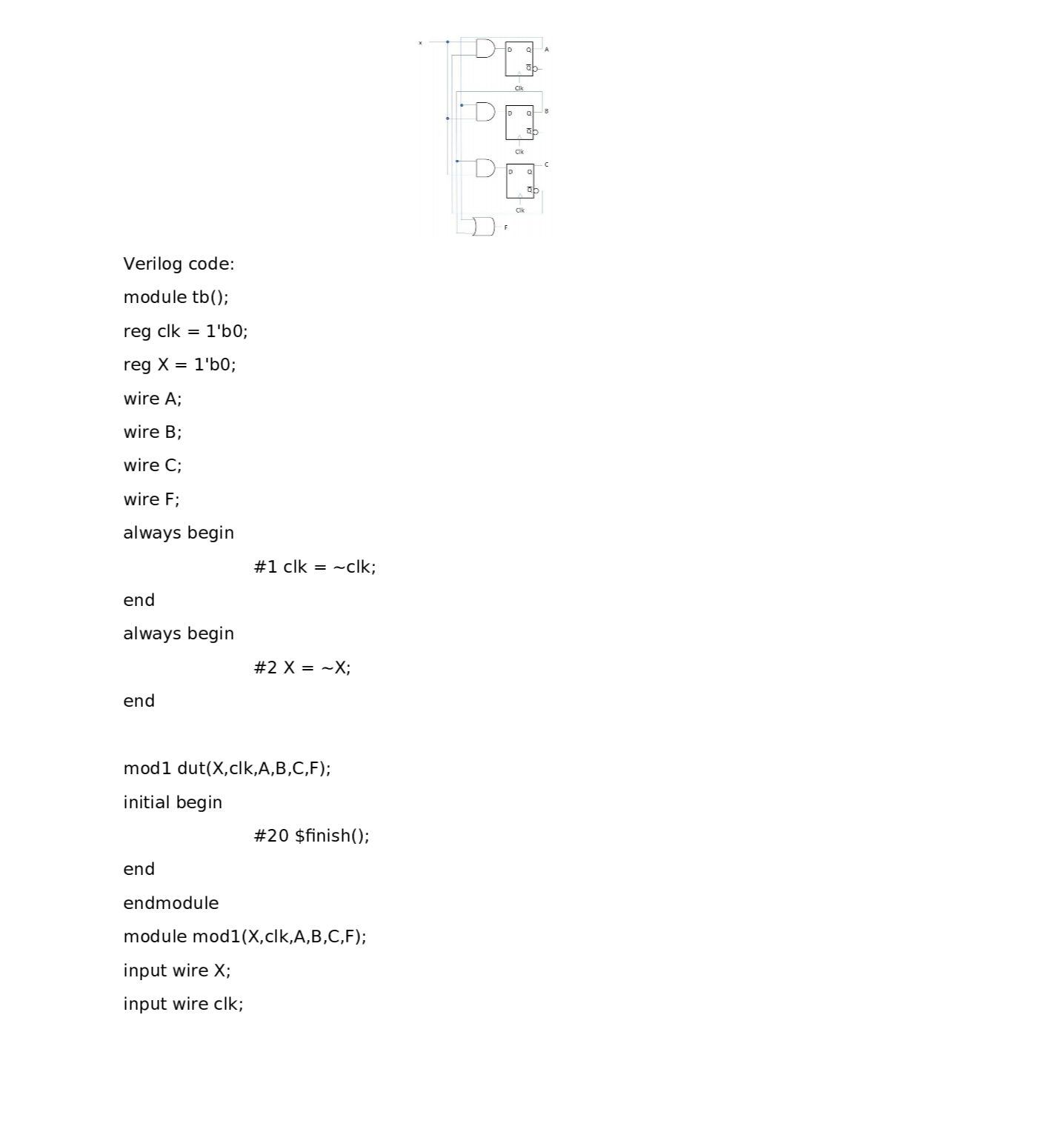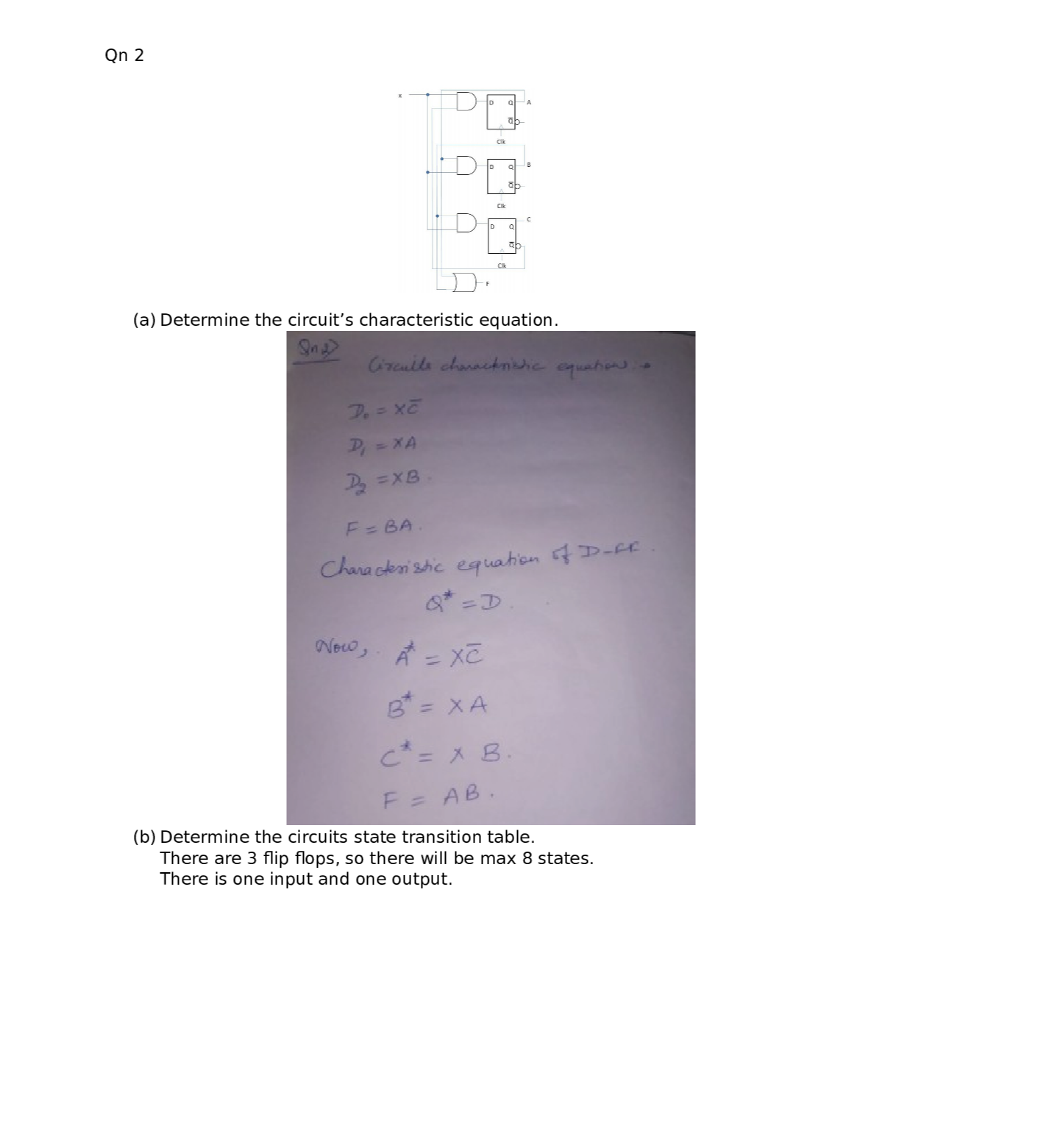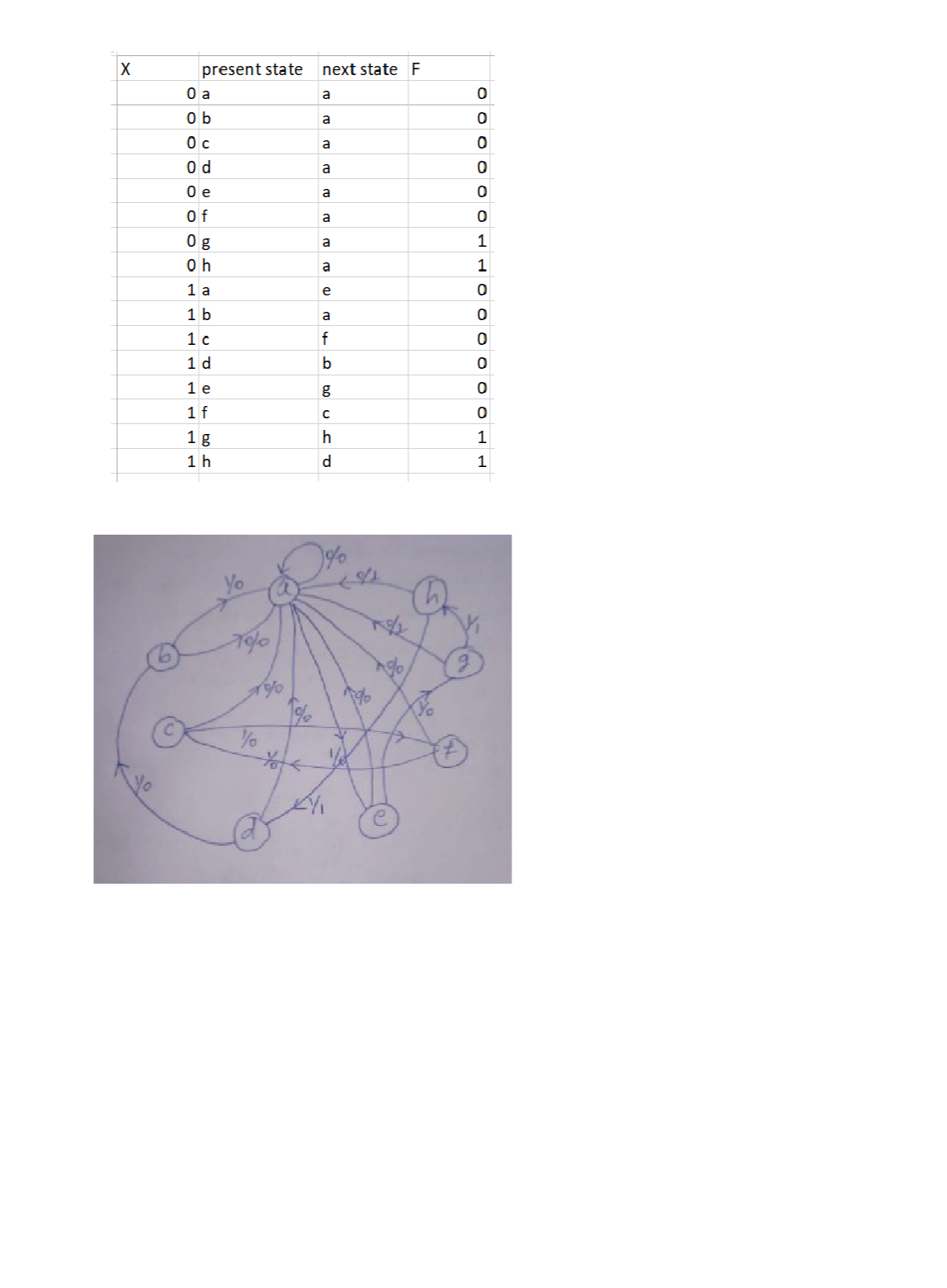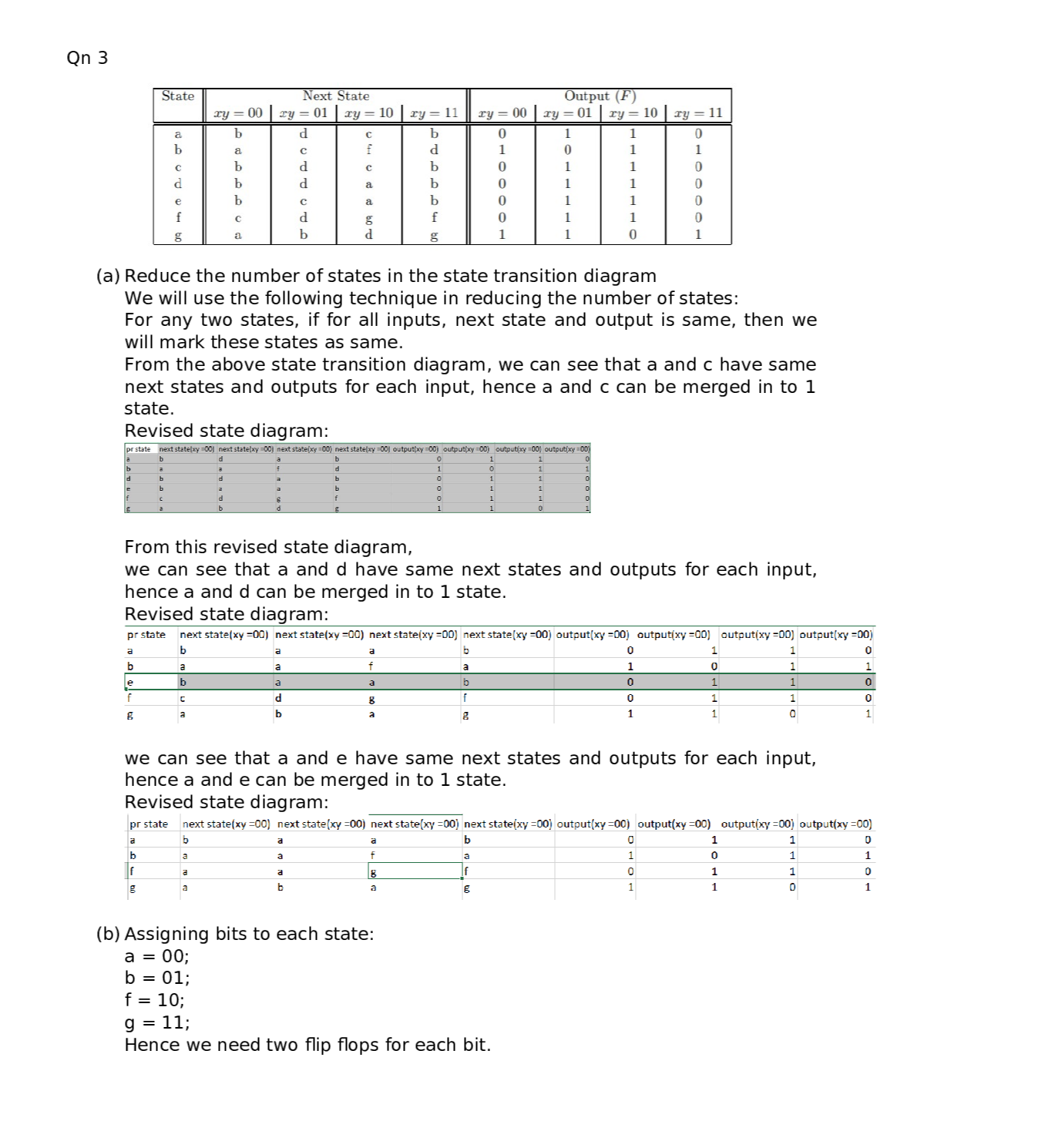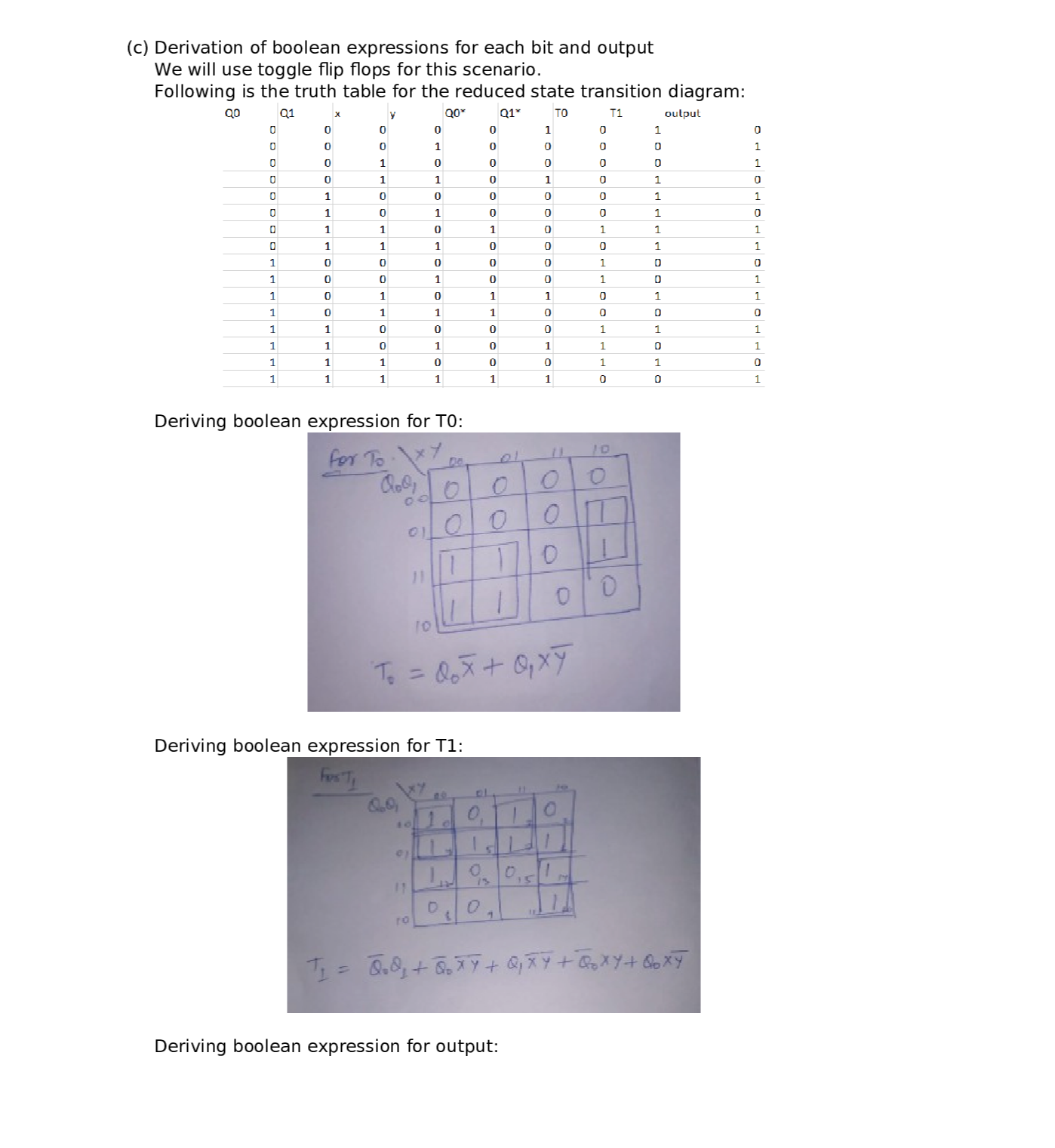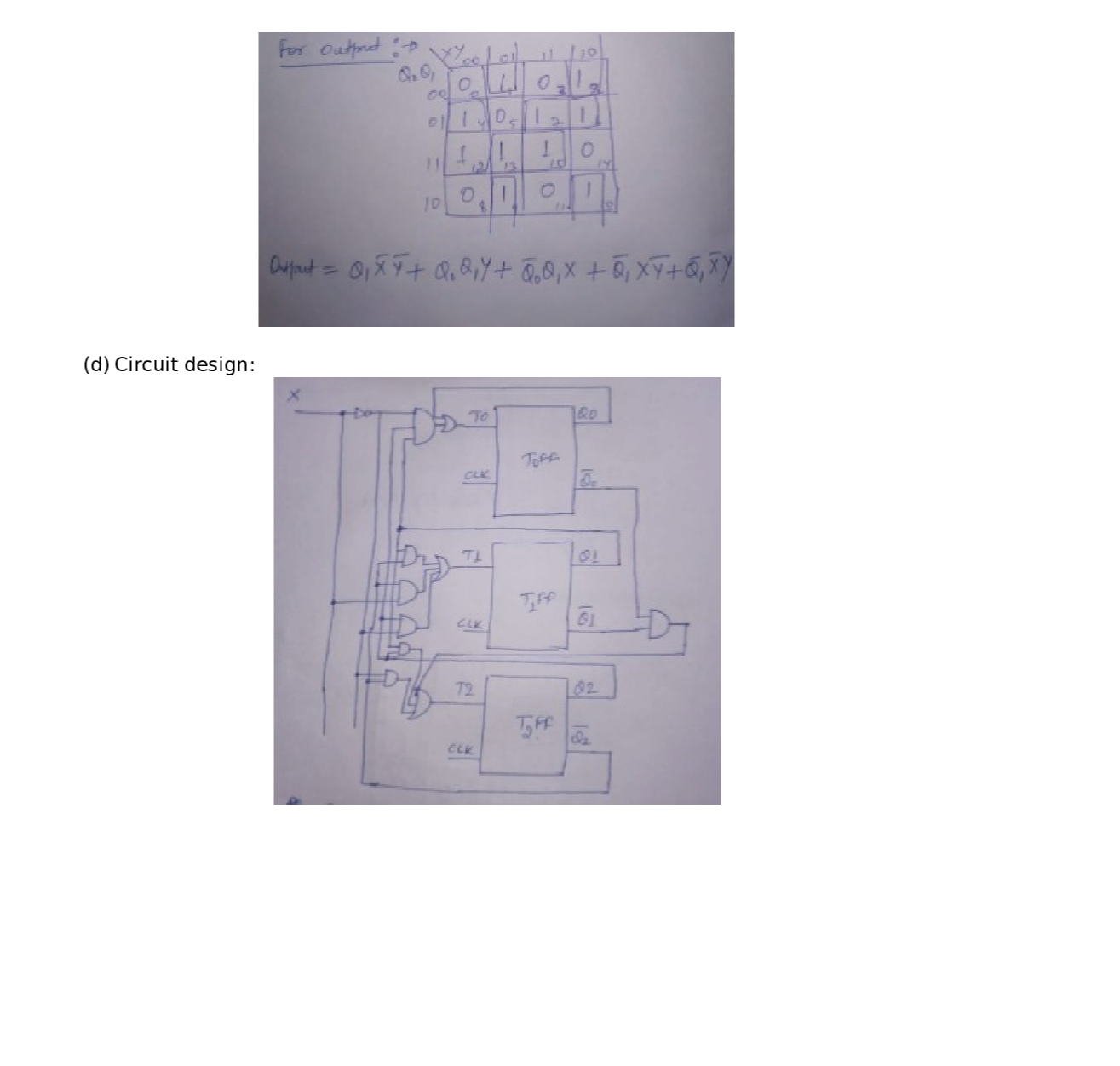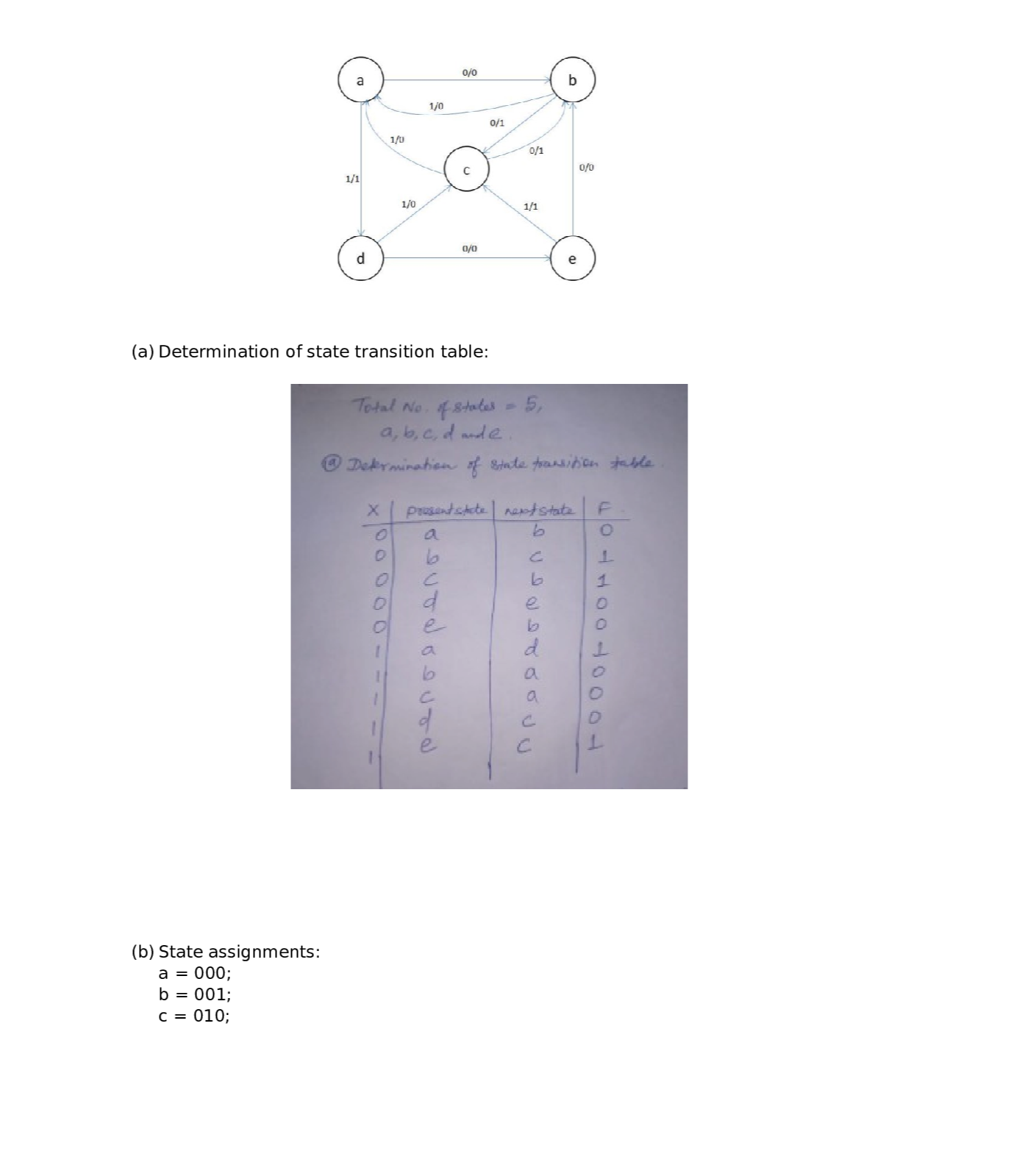### Question 52583Circuits

Problem 3 For the following circuit, use nodal analysis to write the FOUR equations in standard form. DO NOT SOLVE. You must identify any quasi-supernodes, supernodes, auxiliary equations, and remove dependent variables. Your final equations may only have V₁, V2, V3 and/or V4 as unknowns. Put your final equations in standard form in the box provided.

### Question 52582Circuits

Problem 2 For the following circuit, write the FOUR mesh equations in standard form. DO NOT SOLVE. You must identify any super meshes, auxiliary equations, and re-move all dependent variables. Your equations may only have 11,12,13 and/or 14 as unknowns. Put your final equations in standard form in the box provided.

### Question 52581Circuits

Problem 1.1 Determine Reg in Ohms at the (a,b) terminals for the following circuit. Round your answer to the nearest single digit decimal place (tenths).
Problem 1.2 Determine the voltage V, in Volts for the following circuit. Round your answer to the nearest single digit decimal place (tenths).
Problem 1.3 Determine the current I, in Amps for the following circuit. Round your answer to the nearest single digit decimal place (tenths).
Problem 1.4 Determine the power, P3n, delivered to the 302 resistor in Watts for the following circuit.Round your answer to the nearest single digit decimal place (tenths).

### Question 45529Circuits

11) From your answer in (10), does the 4V 'DC' or steady state voltage impact the value of the displacement current, Ic.

### Question 45528Circuits

\text { 10) Assuming } I_{C}=C \frac{d V_{C}}{d t} \text {, find the displacement current for } V_{C}=e^{-\omega t}+4 V \text { and } V_{C}=
sin(wt) + 4 V.

### Question 45527Circuits

9) Based on your answer in (7), can you apply an arbitrarily large field or voltage across a dielectric? What is the name for this limitation and what is its value for Air and Glass (SiO2)?

### Question 45526Circuits

8) Remembering quantum tunneling from Chapter 3, what is happening to the probability of a tunneling event as the "thickness" of the barrier representing the dielectric decreases?

### Question 45525Circuits

7) What happens to the relative "thickness", or distance an electron must travel in a straight line across the dielectric, as you increase the voltage?

### Question 45524Circuits

6) Redraw the diagram in (5) with a small positive voltage on the right-hand side (no voltage or OVon the left-hand side).

### Question 45523Circuits

5) Draw the energy band diagram of a capacitor made of two aluminum plates with a glass dielectric. The capacitor should have zero applied voltage.

### Submit query

Getting answers to your urgent problems is simple. Submit your query in the given box and get answers Instantly.

### Submit a new QuerySuccess

Assignment is successfully created